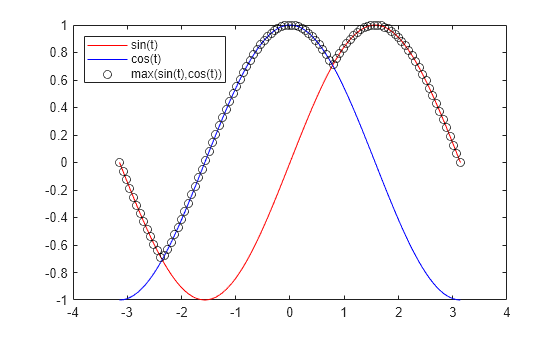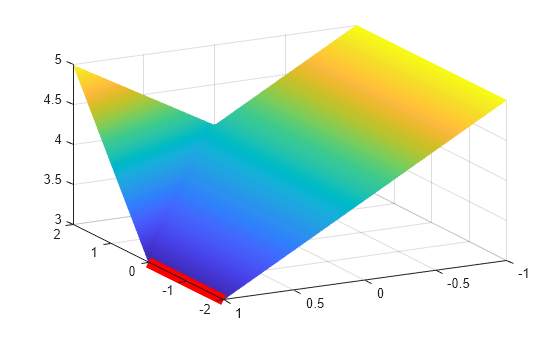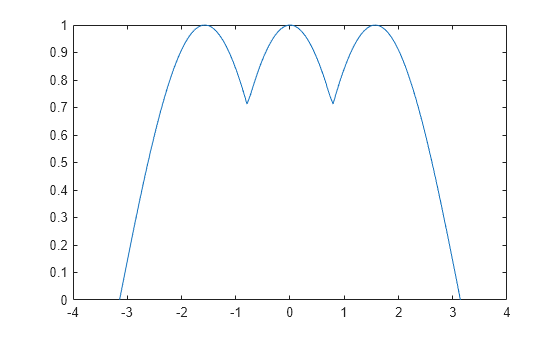# fminimax

## 语法

``x = fminimax(fun,x0)``
``x = fminimax(fun,x0,A,b)``
``x = fminimax(fun,x0,A,b,Aeq,beq)``
``x = fminimax(fun,x0,A,b,Aeq,beq,lb,ub)``
``x = fminimax(fun,x0,A,b,Aeq,beq,lb,ub,nonlcon)``
``x = fminimax(fun,x0,A,b,Aeq,beq,lb,ub,nonlcon,options)``
``x = fminimax(problem)``
``[x,fval] = fminimax(___)``
``[x,fval,maxfval,exitflag,output] = fminimax(___)``
``[x,fval,maxfval,exitflag,output,lambda] = fminimax(___)``

## 说明

`fminimax` 寻找能够最小化一组目标函数最大值的点。

x、lb 和 ub 可以作为向量或矩阵传递；请参阅矩阵参数

`$\underset{x}{\mathrm{max}}\underset{i}{\mathrm{min}}{F}_{i}\left(x\right)=-\underset{x}{\mathrm{min}}\underset{i}{\mathrm{max}}\left(-{F}_{i}\left(x\right)\right).$`

`$\underset{x}{\mathrm{min}}\underset{i}{\mathrm{max}}|{F}_{i}\left(x\right)|$`

（求解过程中需要使用 `AbsoluteMaxObjectiveCount` 选项。）请参阅使用一个目标的绝对值求解 minimax 问题

````x = fminimax(fun,x0)` 从 `x0` 开始，求 `fun` 中所述的函数的 minimax 解 `x`。 注意传递额外参数说明如何将额外的参数传递给目标函数和非线性约束函数（如有必要）。 ```

``x = fminimax(fun,x0,A,b)` 在满足线性不等式 `A*x ≤ b` 的情况下求解 minimax 问题。`
``x = fminimax(fun,x0,A,b,Aeq,beq)` 进一步在满足线性等式 `Aeq*x = beq` 的情况下求解 minimax 问题。如果不存在不等式，则设置 `A = []` 和 `b = []`。`

````x = fminimax(fun,x0,A,b,Aeq,beq,lb,ub)` 在满足边界 `lb `≤` x `≤` ub` 的情况下求解 minimax 问题。如果不存在等式，请设置 `Aeq = []` 和 `beq = []`。如果 `x(i)` 无下界，则设置 `lb(i) = –Inf`；如果 `x(i)` 无上界，则设置 `ub(i) = Inf`。 注意请参阅迭代可能违反约束。 注意如果为问题指定的输入边界不一致，则输出 `x` 为 `x0`，输出 `fval` 为 `[]`。 ```

``x = fminimax(fun,x0,A,b,Aeq,beq,lb,ub,nonlcon)` 在满足 `nonlcon` 中定义的非线性不等式 `c(x)` 或等式 `ceq(x)` 的情况下求解 minimax 问题。该函数会进行优化，以满足 `c(x) ≤ 0` 和 `ceq(x) = 0`。如果不存在边界，则设置 `lb = []` 和/或 `ub = []`。`

``x = fminimax(fun,x0,A,b,Aeq,beq,lb,ub,nonlcon,options)` 使用在 `options` 中指定的优化选项求解 minimax 问题。使用 `optimoptions` 可设置这些选项。`
``x = fminimax(problem)` 求解 `problem` 的 minimax 问题，它是 `problem` 中所述的一个结构体。`

``[x,fval] = fminimax(___)` 对上述任何语法，返回目标函数 `fun` 在解 `x` 处计算的值。`

``[x,fval,maxfval,exitflag,output] = fminimax(___)` 还返回解 `x` 处目标函数的最大值、一个描述 `fminimax` 退出条件的值 `exitflag`，以及一个包含优化过程信息的结构体 `output`。`

``[x,fval,maxfval,exitflag,output,lambda] = fminimax(___)` 还返回结构体 `lambda`，其字段包含在解 `x` 处的拉格朗日乘数。`

## 示例

```t = linspace(-pi,pi); plot(t,sin(t),'r-') hold on plot(t,cos(t),'b-'); plot(t,max(sin(t),cos(t)),'ko') legend('sin(t)','cos(t)','max(sin(t),cos(t))','Location','NorthWest')``````fun = @(x)[sin(x);cos(x)]; x0 = 1; x1 = fminimax(fun,x0)```
```Local minimum possible. Constraints satisfied. fminimax stopped because the size of the current search direction is less than twice the value of the step size tolerance and constraints are satisfied to within the value of the constraint tolerance. ```
```x1 = 0.7854 ```

```x0 = -2; x2 = fminimax(fun,x0)```
```Local minimum possible. Constraints satisfied. fminimax stopped because the size of the current search direction is less than twice the value of the step size tolerance and constraints are satisfied to within the value of the constraint tolerance. ```
```x2 = -2.3562 ```

```a = [1;1]; b = [-1;1]; c = [0;-1]; a0 = 2; b0 = -3; c0 = 4; fun = @(x)[x*a+a0,x*b+b0,x*c+c0];```

```A = [1,3]; b = -4; x0 = [-1,-2]; x = fminimax(fun,x0,A,b)```
```Local minimum possible. Constraints satisfied. fminimax stopped because the size of the current search direction is less than twice the value of the step size tolerance and constraints are satisfied to within the value of the constraint tolerance. ```
```x = 1×2 -5.8000 0.6000 ```

```a = [1;1]; b = [-1;1]; c = [0;-1]; a0 = 2; b0 = -3; c0 = 4; fun = @(x)[x*a+a0,x*b+b0,x*c+c0];```

```lb = [-2,-1]; ub = [2,1]; x0 = [0,0]; A = []; % No linear constraints b = []; Aeq = []; beq = []; [x,fval] = fminimax(fun,x0,A,b,Aeq,beq,lb,ub)```
```Local minimum possible. Constraints satisfied. fminimax stopped because the size of the current search direction is less than twice the value of the step size tolerance and constraints are satisfied to within the value of the constraint tolerance. ```
```x = 1×2 -0.0000 1.0000 ```
```fval = 1×3 3.0000 -2.0000 3.0000 ```

```[X,Y] = meshgrid(linspace(-2,2),linspace(-1,1)); Z = max(fun([X(:),Y(:)]),[],2); Z = reshape(Z,size(X)); surf(X,Y,Z,'LineStyle','none') view(-118,28) hold on line([-2,0],[1,1],[3,3],'Color','r','LineWidth',8) hold off``````a = [1;1]; b = [-1;1]; c = [0;-1]; a0 = 2; b0 = -3; c0 = 4; fun = @(x)[x*a+a0,x*b+b0,x*c+c0];```

`unitdisk` 函数表示非线性不等式约束 $‖x{‖}^{2}\le 1$

`type unitdisk`
```function [c,ceq] = unitdisk(x) c = x(1)^2 + x(2)^2 - 1; ceq = []; ```

`x0 = [0,0]` 开始，在 `unitdisk` 约束下求解 minimax 问题。

```x0 = [0,0]; A = []; % No other constraints b = []; Aeq = []; beq = []; lb = []; ub = []; nonlcon = @unitdisk; x = fminimax(fun,x0,A,b,Aeq,beq,lb,ub,nonlcon)```
```Local minimum possible. Constraints satisfied. fminimax stopped because the size of the current search direction is less than twice the value of the step size tolerance and constraints are satisfied to within the value of the constraint tolerance. ```
```x = 1×2 -0.0000 1.0000 ```

```fun = @(x)[sin(x),cos(x)]; options = optimoptions('fminimax','AbsoluteMaxObjectiveCount',1); x0 = 1; A = []; % No constraints b = []; Aeq = []; beq = []; lb = []; ub = []; nonlcon = []; x1 = fminimax(fun,x0,A,b,Aeq,beq,lb,ub,nonlcon,options)```
```Local minimum possible. Constraints satisfied. fminimax stopped because the size of the current search direction is less than twice the value of the step size tolerance and constraints are satisfied to within the value of the constraint tolerance. ```
```x1 = 0.7854 ```

`x0 = –2` 开始尝试。

```x0 = -2; x2 = fminimax(fun,x0,A,b,Aeq,beq,lb,ub,nonlcon,options)```
```Local minimum possible. Constraints satisfied. fminimax stopped because the size of the current search direction is less than twice the value of the step size tolerance and constraints are satisfied to within the value of the constraint tolerance. ```
```x2 = -3.1416 ```

```t = linspace(-pi,pi); plot(t,max(abs(sin(t)),cos(t)))``````a = [1;1]; b = [-1;1]; c = [0;-1]; a0 = 2; b0 = -3; c0 = 4; fun = @(x)[x*a+a0,x*b+b0,x*c+c0];```

```x0 = [0,0]; [x,fval] = fminimax(fun,x0)```
```Local minimum possible. Constraints satisfied. fminimax stopped because the size of the current search direction is less than twice the value of the step size tolerance and constraints are satisfied to within the value of the constraint tolerance. ```
```x = 1×2 -2.5000 2.2500 ```
```fval = 1×3 1.7500 1.7500 1.7500 ```

```a = [1;1]; b = [-1;1]; c = [0;-1]; a0 = 2; b0 = -3; c0 = 4; fun = @(x)[x*a+a0,x*b+b0,x*c+c0];```

```A = [1,3]; b = -4; x0 = [-1,-2];```

```options = optimoptions('fminimax','Display','iter'); Aeq = []; % No other constraints beq = []; lb = []; ub = []; nonlcon = []; [x,fval,maxfval,exitflag,output,lambda] =... fminimax(fun,x0,A,b,Aeq,beq,lb,ub,nonlcon,options)```
``` Objective Max Line search Directional Iter F-count value constraint steplength derivative Procedure 0 4 0 6 1 9 5 0 1 0.981 2 14 4.889 8.882e-16 1 -0.302 Hessian modified twice 3 19 3.4 8.132e-09 1 -0.302 Hessian modified twice Local minimum possible. Constraints satisfied. fminimax stopped because the size of the current search direction is less than twice the value of the step size tolerance and constraints are satisfied to within the value of the constraint tolerance. ```
```x = 1×2 -5.8000 0.6000 ```
```fval = 1×3 -3.2000 3.4000 3.4000 ```
```maxfval = 3.4000 ```
```exitflag = 4 ```
```output = struct with fields: iterations: 4 funcCount: 19 lssteplength: 1 stepsize: 6.0684e-10 algorithm: 'active-set' firstorderopt: [] constrviolation: 8.1323e-09 message: 'Local minimum possible. Constraints satisfied....' ```
```lambda = struct with fields: lower: [2x1 double] upper: [2x1 double] eqlin: [0x1 double] eqnonlin: [0x1 double] ineqlin: 0.2000 ineqnonlin: [0x1 double] ```

• 两个目标函数值在解处相等。

• 求解器在经过 4 次迭代和 19 次函数计算后收敛。

• `lambda.ineqlin` 值非零，表示线性约束在解处为活动状态。

## 输入参数

`x = fminimax(@myfun,x0,goal,weight)`

```function F = myfun(x) F = ... % Compute function values at x.```

`fun` 也可以是匿名函数的函数句柄：

`x = fminimax(@(x)sin(x.*x),x0,goal,weight);`

`fminimax``x0` 参数的形状将 `x` 传递给目标函数和任何非线性约束函数。例如，如果 `x0` 是 5×3 数组，则 `fminimax``x` 以 5×3 数组的形式传递给 `fun`。但是，在将 `x` 转换为列向量 `x(:)` 后，`fminimax` 会将线性约束矩阵 `A``Aeq` 乘以 `x`

`options = optimoptions('fminimax','SpecifyObjectiveGradient',true)`

`A` 以如下形式编写 `M` 个线性不等式

`A*x <= b`,

x1 +2x2 ≤10
3x1 +4x2 ≤20
5x1 +6x2 ≤30，

```A = [1,2;3,4;5,6]; b = [10;20;30];```

`b` 以如下形式编写 `M` 个线性不等式

`A*x <= b`,

x1 + 2x2 ≤ 10
3x1 + 4x2 ≤ 20
5x1 + 6x2 ≤ 30。

```A = [1,2;3,4;5,6]; b = [10;20;30];```

`Aeq` 以如下形式编写 `Me` 个线性等式

`Aeq*x = beq`,

x1 +2x2 +3x3 =10
2x1 +4x2 + x3 =20，

```Aeq = [1,2,3;2,4,1]; beq = [10;20];```

`beq` 以如下形式编写 `Me` 个线性等式

`Aeq*x = beq`,

x1 + 2x2 + 3x3 = 10
2x1 + 4x2 + x3 = 20。

```Aeq = [1,2,3;2,4,1]; beq = [10;20];```

`x(i) >= lb(i)`（对于全部 `i`）。

`x(i) >= lb(i)` (`1 <= i <= numel(lb)`)。

`x(i) <= ub(i)`（对于全部 `i`）。

`x(i) <= ub(i)` (`1 <= i <= numel(ub)`)。

• `c(x)` 是由 `x` 处的非线性不等式约束组成的数组。`fminimax` 尝试满足

`c(x) <= 0` for all entries of `c`.

• `ceq(x)` 是由 `x` 处的非线性等式约束组成的数组。`fminimax` 尝试满足

`ceq(x) = 0` for all entries of `ceq`.

`x = fminimax(@myfun,x0,...,@mycon)`

```function [c,ceq] = mycon(x) c = ... % Compute nonlinear inequalities at x. ceq = ... % Compute nonlinear equalities at x.```

`options = optimoptions('fminimax','SpecifyConstraintGradient',true)`

`optimoptions` 显示中缺少某些选项。这些选项在下表中以斜体显示。有关详细信息，请参阅查看优化选项

`AbsoluteMaxObjectiveCount`

`ConstraintTolerance`

Diagnostics

DiffMaxChange

DiffMinChange

`Display`

• `'off'``'none'` 不显示输出。

• `'iter'` 显示每次迭代的输出，并给出默认退出消息。

• `'iter-detailed'` 显示每次迭代的输出，并给出带有技术细节的退出消息。

• `'notify'` 仅当函数不收敛时才显示输出，并给出默认退出消息。

• `'notify-detailed'` 仅当函数不收敛时才显示输出，并给出技术性退出消息。

• `'final'`（默认值）仅显示最终输出，并给出默认退出消息。

• `'final-detailed'` 仅显示最终输出，并给出带有技术细节的退出消息。

`FiniteDifferenceStepSize`

`delta = v.*sign′(x).*max(abs(x),TypicalX);`

`delta = v.*max(abs(x),TypicalX);`

`FiniteDifferenceType`

`FunctionTolerance`

FunValCheck

`MaxFunctionEvaluations`

`MaxIterations`

MaxSQPIter

MeritFunction

`OptimalityTolerance`

`OutputFcn`

`PlotFcn`

• `'optimplotx'` 绘制当前点。

• `'optimplotfunccount'` 绘制函数计数。

• `'optimplotfval'` 绘制目标函数值。

• `'optimplotconstrviolation'` 绘制最大约束违反值。

• `'optimplotstepsize'` 绘制步长。

RelLineSrchBnd

RelLineSrchBndDuration

`RelLineSrchBnd` 所指定的边界应处于活动状态的迭代次数。默认值为 `1`

`SpecifyConstraintGradient`

`SpecifyObjectiveGradient`

`StepTolerance`

`x` 的终止容差（正标量）。默认值为 1e-6。请参阅容差和停止条件

TolConSQP

`TypicalX`

`UseParallel`

`objective`

`x0`

`x` 的初始点

`Aineq`

`bineq`

`Aeq`

`beq`

`lb`由下界组成的向量
`ub`由上界组成的向量

`nonlcon`

`solver`

`'fminimax'`

`options`

`optimoptions` 创建的选项

## 输出参数

`fminimax` 停止的原因，以整数形式返回。

 `1` 函数收敛于解 `x` `4` 搜索方向的模小于指定的容差，约束违反值小于 `options.ConstraintTolerance` `5` 方向导数的模小于指定容差，约束违反值小于 `options.ConstraintTolerance` `0` 迭代次数超过 `options.MaxIterations` 或函数计算次数超过 `options.MaxFunctionEvaluations` `-1` 由输出函数或绘图函数停止 `-2` 找不到可行点。

 `iterations` 执行的迭代次数 `funcCount` 函数计算次数 `lssteplength` 搜索方向上线搜索步的大小 `constrviolation` 约束函数的最大值 `stepsize` `x` 中最后一次位移的长度 `algorithm` 使用的优化算法 `firstorderopt` 一阶最优性的测度 `message` 退出消息

 `lower` 对应于 `lb` 的下界 `upper` 对应于 `ub` 的上界 `ineqlin` 对应于 `A` 和 `b` 的线性不等式 `eqlin` 对应于 `Aeq` 和 `beq` 的线性等式 `ineqnonlin` 对应于 `nonlcon` 中 `c` 的非线性不等式 `eqnonlin` 对应于 `nonlcon` 中 `ceq` 的非线性等式

## 算法

`fminimax` 将 minimax 问题转换为目标达到问题，然后使用 `fgoalattain` 求解转换后的目标达到问题，从而对 minimax 问题求解。转换将所有目标设置为 0，并将所有权重设置为 1。请参阅Multiobjective Optimization Algorithms中的公式 1